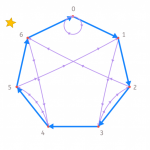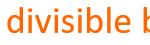Smartick is an advanced online program that teaches kids math and coding in only 15 min. a day

Apr06

## The Reason for Divisibility CriteriaSometimes we may be interested in knowing if one number is divisible by another, without having to perform a division. This is especially useful when we work with large numbers that require a lot of time for completing calculations. If you would like to refresh your memory on divisibility criteria, take a look at this […]

Dec31

## Divisibility by 4: How Do We Know If a Number Is Divisible by 4?In this post, we are going to learn the criteria for divisibility of the number 4 and understand how they function. The divisibility criteria for the number 4 are rules to know if a number can be divided by 4. They are simple to learn and their explanations are easy to understand. How do we […]

Aug07

## Divisibility Guidelines for 5 and Some ExamplesIn this post we will learn the criteria of divisibility by 5. A number is divisible by 5 when dividing the number by 5 results in a remainder of zero. But how can we know without having to complete the division? Here we show you the easiest way.   Divisibility criteria for 5: To determine if a number is […]

Jun07

## Follow the Divisibility Guidelines for 3In the post for this week, I will explain the criteria for the divisibility of 3. When they ask you “Is this number divisible by 3?”  The most intuitive thing to do is to divide that number by 3, and if the remainder is zero then the number itself is divisible by 3. For example: One […]

May10

## Divisibility Criteria from 2 to 13 and an ExampleThe criteria for divisibility are guidelines that help us to quickly know if a number is divisible or not. In other words, it lets us know if a number divides evenly or not. The criteria for divisibility are very useful. They help us easily find the divisors of a given number. They are especially helpful […]